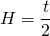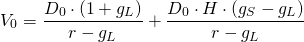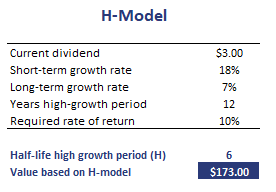# H-Model

For many companies, we expect the growth rate of earning to decrease over time as competitors enter the market. Typically, the growth rate does not drop to a low level all at once. instead, the growth rate is likely to decrease over time. The H-model is a variation to the standard dividend growth model first proposed by Myron J. Gordon to model a declining growth rate.  In particular, the H model can be used to model a linearly declining growth rate.

On this page, we discuss the H model definition, provide the H-model formula and finally implement the H-model in Excel. The dividend growth model calculator is available at the bottom of the page.

## H-Model definition

In most cases, the long-term growth rate does not drop abruptly from the high growth rate to the low growth rate. Instead, we can imagine that the growth rate slowly declines to the long-term growth rate. This can easily be accomplished using the H-model.

Before we continue, there is one important aspect we need to stress. The H-model is an approximation. That means that the result will not be exactly equal to the value if we model each of the cash flows separately. The approximation will be more accurate

• the shorter the high-growth period
• the smaller the spread between the short-term and the long-term growth rate

The H in the H-model refers to a particular parameter that we have to calculate. In particular, H is the half-life of the model. This parameter captures the speed at which the growth rate converts to the long-term growth rate. It is calculated as followswhere t is the length of the high growth period.

## H-model formula

Once we have the H, the full H-model formula looks as follows:where D0 is the current dividend, gS is the short-term growth rate, gL is the long-term growth rate, and r is the required rate of return.

## H-model interpretation

The first term is what the shares would be would be worth if there was only a low growth period. The second term is an approximation of the value that results from the linearly declining high-growth phase.

## H model example

Finally, let’s put the formulas to work. The following table implements the above formulae to calculate the fundamental value of a growth company. The spreadsheet can be downloaded using the link reported below.## Summary

We discussed the H-model, a simple formula to approximate the value of a company for which we expect the high growth rate to decline linearly to a lower long-term growth rate.

### H-model calculator Excel

Want to have an implementation in Excel? Download the Excel file: H-model implementation